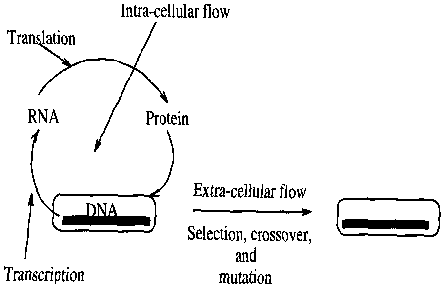# Difference between revisions of "Genetic algorithm"

Genetic algorithms [a1], [a2], [a3] describe a class of stochastic search algorithms that are intended to work by processing relations (called partitions in the genetic algorithms literature) and classes (schemata). Genetic algorithms are used in search, optimization, and machine learning for extremizing the objective function, when little domain knowledge is available. Genetic algorithms simultaneously sample from different regions of the search space and try to decide about better regions of the search space. This is an inductive process, i.e., this is a process of guessing about the unknown from some observed behaviours. Induction is no better than enumeration when relations among the different members of the search space are not exploited. The foundation of genetic algorithms is based on this observation. Genetic algorithms search by processing relations and classes defined by the representation. For example, \$l\$ features of a sequence representation may define \$2^l\$ equivalence relations. Genetic algorithms implicitly process these relations in a very parallel manner and detect good regions of the search space.Figure: g110150a

Information flow in natural evolution

The beauty of genetic algorithms is that the processing of relations and classes is subtly implemented using the biological framework of natural evolution. The evolutionary flow of genetic information is primarily comprised of the extra-cellular and intra-cellular flow of information. Fig.a1 presents a schematic description of the processes. Genetic algorithms are often designed based on the extra-cellular flow of genetic information [a1], [a2] with few exceptions [a4]. The extra-cellular flow is defined by the transmission of DNA from generation to generation through selection, crossover, and mutation. Genetic algorithms use such operators for detecting better relations and classes that finally may lead to the desired solution. The following section describes a simple genetic algorithm [a1], [a2].

## Simple genetic algorithm.

The simple genetic algorithm [a1], [a3] works from a population of samples defined using some representation and searches by selection, crossover, and mutation operators. The sample population is first randomly chosen. This is followed by successive application of each of the above mentioned operators. These components are described below.

The overall algorithm is presented below.

``` ```

```t = 0; // Set generation counter to zero. Initialize(Pop(t)); // Randomly initialize Evaluate(Pop(t)); // Evaluate fitness Repeat { Selection(Pop(t)); // Select good ones Crossover(Pop(t)); // Cross strings Mutation(Pop(t)); // Mutate strings Evaluate(Pop(t)); // Evaluate fitness t = t + 1; // Increment counter } Until (termination condition = TRUE) // A pseudo-code for simple genetic algorithm ```

### Population.

The simple genetic algorithm often uses a sequence representation. A string usually represents a unique member of the search space. Following the biological analogy, strings are sometimes called chromosomes. Every chromosome is a sequence of genes. Every gene takes a value from the alphabet set of the chosen representation. The population is a collection of such strings.

### Selection.

The selection operator is responsible for detecting better regions of the search space. The "fitness" of a member is its objective function value. Selection computes an ordering among all the members of the population and gives more copies to the better strings at the expense of less "fit" members. There exist various kinds of different tools of selection operators, such as roulette wheel and tournament selection. Selection alone can lead the population to convergence in polynomial time.

### Crossover.

Crossover works by swapping portions between two strings. Single-point crossover [a2] is often used in the simple genetic algorithm. It works by first randomly picking a point between \$0\$ and \$l\$. The participating strings are then split at that point, followed by a swapping of the split halves. In absence of any bias, uniform application of crossover simply decorrelates the individual distribution of genes in the population. However, crossover can be effectively used for computing set intersections when properly guided by particular relations [a4]. In the simple genetic algorithm, crossover is used with high probability.

### Mutation.

Mutation randomly changes the entries of a string. Mutation is usually treated as a low profile operator in a genetic algorithm because blind mutation cannot make an algorithm transcend the limits of random enumerative search.

How to Cite This Entry:
Genetic algorithm. Encyclopedia of Mathematics. URL: http://encyclopediaofmath.org/index.php?title=Genetic_algorithm&oldid=11901
This article was adapted from an original article by H. Kargupta (originator), which appeared in Encyclopedia of Mathematics - ISBN 1402006098. See original article